Mathematical and Physical Journal
for High Schools
Issued by the MATFUND Foundation
 Already signed up? New to KöMaL?

# KöMaL Problems in Physics, February 2012

Show/hide problems of signs:## Problems with sign 'M'

Deadline expired on March 12, 2012.

M. 321. Measure the torsional stiffness of the parts of a skewer of different lengths such as 10, 15 and 20 cm (torsional stiffness is the ratio of applied torsion moment to angle of twist) a) with torsional swings, b) with static method.

(6 pont)

statistics## Problems with sign 'P'

Deadline expired on March 12, 2012.

P. 4413. Every morning two runners run along the running track of a stadium. If they run in the same direction 11 times more time elapses between the two moments when they meet then when they run in the opposite direction. (Their speeds are constant.) What is the ratio of the speeds of the two runners?

(3 pont)

solution (in Hungarian), statistics

P. 4414. Is it true that the attractive force exerted by the Earth on the Moon is less than that of exerted by the Sun on the Moon? If yes, why does the Moon rotate about the Earth instead of about the Sun?

(3 pont)

solution (in Hungarian), statistics

P. 4415. A sample of water is initially has a temperature of 14 oC. It is heated to its boiling point at atmospheric pressure, and then it is boiled down. What per cent of the added thermal energy is the work done by the expanding gas?

(4 pont)

solution (in Hungarian), statistics

P. 4416. A train travels at a speed of v0, then it slows down uniformly during a time of T, and then it stops. In one of the wagons there is a small object on the floor. How much distance does this small object cover on the floor, and how long does it move? The coefficient of friction is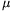.

(5 pont)

solution (in Hungarian), statistics

P. 4417. The best vacuum which can be created in a laboratory at room temperature is 10-10 Pa. How many molecules are there in 1 cm3 of this void''? (In the interstellar medium there is 1 proton in each cm3)

(4 pont)

solution (in Hungarian), statistics

P. 4418. One end of a thin L-shaped tube is sealed, and the tube is held in the vertical plane. The mercury column of length H/2 confines some air (as shown in Figure 1.), the temperature of the confined air is T1=279 K. The external air pressure p0 is equal to the hydrostatic pressure of a mercury column of height H=76 cm. a) Determine the pressure of the confined air.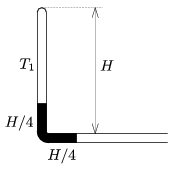Figure 1

b) The tube is turned by 90o, and the air is cooled down such that the air is in the horizontal arm of the L-shaped tube as shown in Figure 2. What is the temperature T2 of the air now?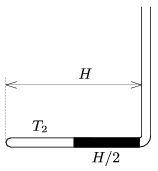Figure 2

(4 pont)

solution (in Hungarian), statistics

P. 4419. A beam of electrons of speed v0 enters into a region of uniform magnetic field of induction B, perpendicularly to the magnetic induction, as shown in the figure. The region of magnetic field has a circular cross section of radius R. Passing the magnetic field, at most what angle do the electrons of the beam deflect with respect to their original path? (Suppose that the magnetic field is weak.)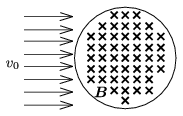(5 pont)

solution (in Hungarian), statistics

P. 4420. The root-mean-square (r.m.s.) value of the voltage of the 50 Hz mains is 230 V. The r.m.s. value of the voltage across a condenser of a series RLC circuit is 100 V, and that of across the ohmic resistor is 200 V. The r.m.s. value of the current in the circuit is 200 mA. What is the relative permeability constant of the iron core of the coil, if the length of the coil is 8 cm, its cross section is 4 cm2 and its number of turns is 300?

(5 pont)

solution (in Hungarian), statistics

P. 4421. An object is moved at a speed of v along the principal axis of a converging lens. What is the speed of the moving image, when the enlargement is N?

(4 pont)

solution (in Hungarian), statistics

P. 4422. A pion (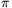+) is a subatomic particle the mass of which is 273 times as big as that of an electron. One of its possible decay mode is a decay into a positron (e+) and an electron-neutrino (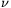e):. What is the minimum speed of that pion which decays such that the positron and the neutrino will have perpendicular velocities? (Assume that the rest mass of the neutrino is 0, so it is a particle for which the relation between its energy and its linear momentum is E=pc.)

(6 pont)

solution (in Hungarian), statistics